# What is a folding square?

Shop for Folding Squares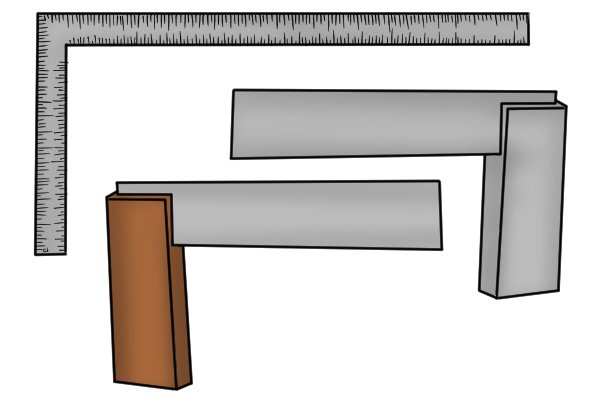Traditionally, squares are measuring devices that have two straight edges fixed at a right angle to one another. They are used for checking the accuracy of right angles as well as measuring and marking lines on a workpiece.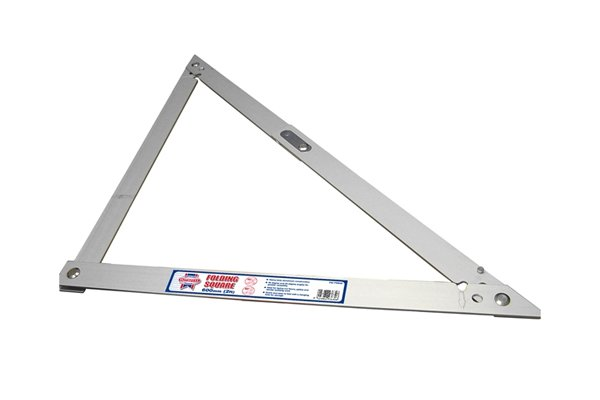A folding square is used for the same purpose, but unlike a regular square, it is able to fold away into one length in order to be stored more easily.

Folding squares are also much larger in size compared to regular squares, making them suitable for large scale projects.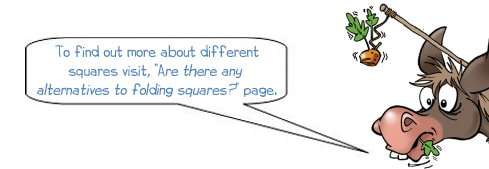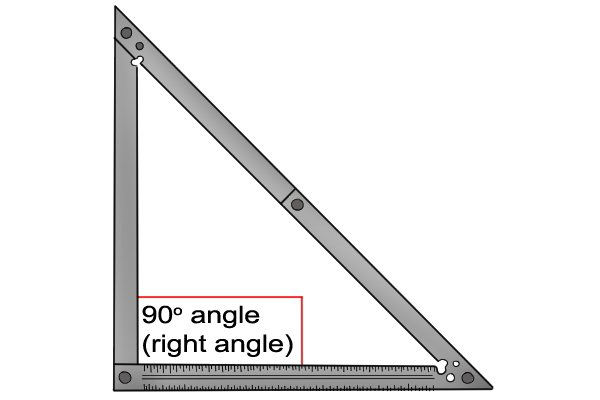The tool is called a ‘folding square’ because it conveniently folds away and because it contains a 90 degree angle (a square angle).

Please note: A folding square is not actually square; it is in fact triangular.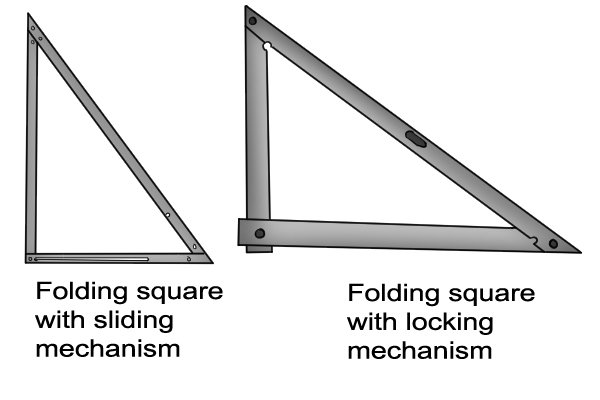There are two types of folding squares, a sliding folding square and a folding square with a locking mechanism.

These mechanisms are used to lock the squares in place and when they are unlocked, allow the frame to start collapse for ease of storage.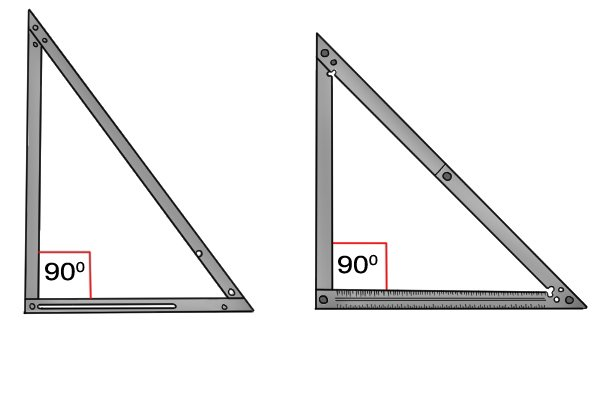All folding squares consist of three angles, one of which will always be 90 degrees (a right angle).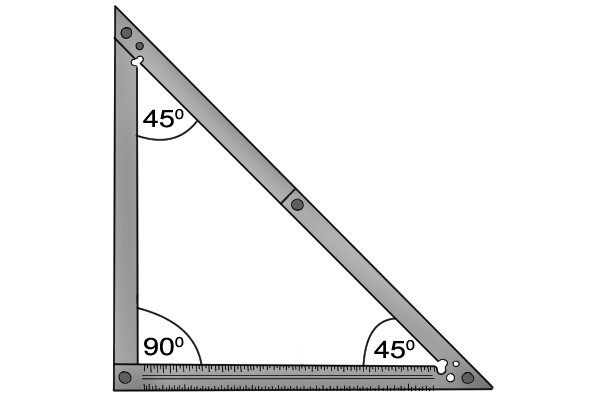Some folding squares contain two 45° angles and one 90° angle.

In mathematical terms, this would be known as an isosceles triangle, two equal sides and two equal angles.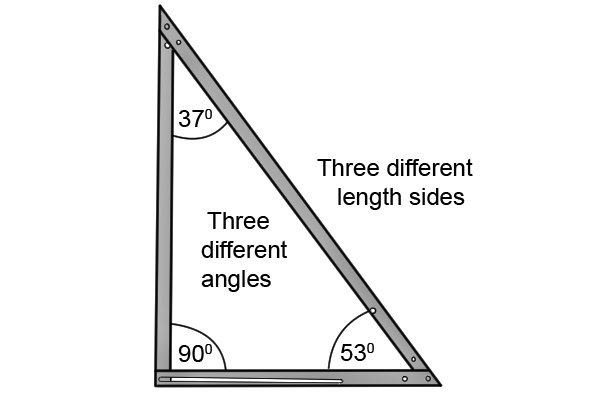Other types of folding squares come with one 90° angle and two different angles of 37° and 53°. These are known as scalene triangles: no sides or angles are equal.

The 37° and 53° angles are not used as they have no significance to the applications that folding squares are used for. However, these squares are still used over regular ones due to their larger size.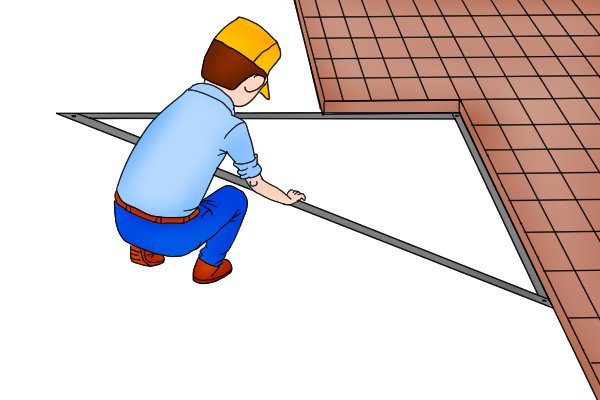Folding squares can be used by professionals or DIYers on site or for jobs at home.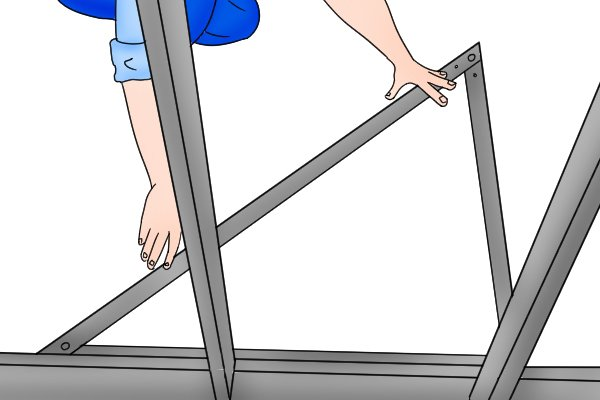They can be used for a number of applications including the laying out of flooring, paving, framing, checking if objects are square and many more.

To find out more about what the folding squares are used for visit, What are folding squares used for?